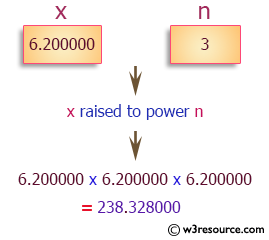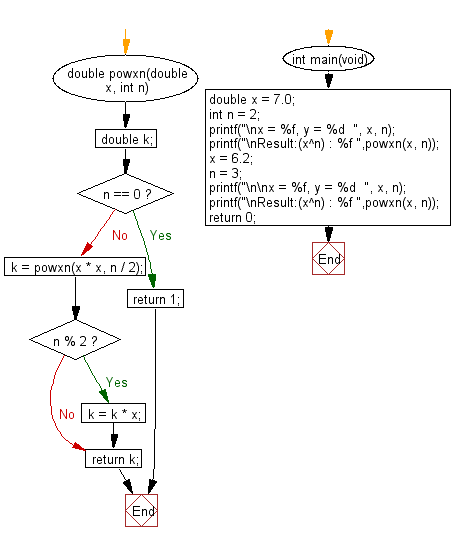﻿ C Program: Calculate x raised to the power n - w3resource

# C Exercises: Calculate x raised to the power n

## C Programming Mathematics: Exercise-4 with Solution

Write a C program to calculate x raised to the power n (xn).

Example:
Input:
x = 7.0
n = 2

x = 6.2
n = 3
Output:
Result:(x^n) : 49.000000
Result:(x^n) : 238.328000

Pictorial Presentation:Sample Solution:

C Code:

``````#include <stdio.h>
#include <limits.h>

double powxn(double x, int n) {
double k;
if (n == 0) return 1;
k = powxn(x * x, n / 2);
if (n % 2) k = k * x;
return k;
}

int main(void)
{
double x = 7.0;
int n = 2;
printf("\nx = %f, y = %d  ", x, n);
printf("\nResult:(x^n) : %f ",powxn(x, n));
x = 6.2;
n = 3;
printf("\n\nx = %f, y = %d  ", x, n);
printf("\nResult:(x^n) : %f ",powxn(x, n));
return 0;
}
```
```

Sample Output:

```x = 7.000000, y = 2
Result:(x^n) : 49.000000

x = 6.200000, y = 3
Result:(x^n) : 238.328000
```

Flowchart:C Programming Code Editor:

Improve this sample solution and post your code through Disqus.

What is the difficulty level of this exercise?

Test your Programming skills with w3resource's quiz.

﻿